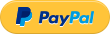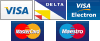Skip to main content# Hydra Fuel Cost Calculator

 Hydra Fuel Cost Calculator Select Miles or Kilometres: Miles Kilometres Calculate the journey cost Calculate the consumption Total Spend = Fuel Cost = Fuel Cost = Trip Distance = Trip Distance = Results Results Examples Compare the difference in costs: